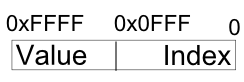# Introduction

This application is a D port of an attempt to resolve an exercise that was posted online by a well known game studio, which was originally written in C++.

The idea behind the port was to learn how D could be used to write such exercise, while at the same time learn the language.

Some care was taken to write good D code, like following D's coding conventions and use of contracts for validation.

If any D community member feels some things could still be improved, please let me know.

# Challenge

The original challenge was as follows:

The problem is to write a set of functions to manage a variable number of byte queues, each with variable length, in a small, fixed amount of memory. You should provide implementations of the following four functions:

``````Q * createQueue(); //Creates a FIFO byte queue, returning a handle to it.
void destroyQueue(Q * q); //Destroy an earlier created byte queue.
void enqueueByte(Q * q, unsigned char b); //Adds a new byte to a queue.
unsigned char dequeueByte(Q * q); //Pops the next byte off the FIFO queue.
``````

So, the output from the following set of calls:

``````Q * q0 = createQueue();
enqueueByte(q0, 0);
enqueueByte(q0, 1);
Q * q1 = createQueue();
enqueueByte(q1, 3);
enqueueByte(q0, 2);
enqueueByte(q1, 4);
printf("%d ", dequeueByte(q0));
printf("%d\n", dequeueByte(q0));
enqueueByte(q0, 5);
enqueueByte(q1, 6);
printf("%d ", dequeueByte(q0));
printf("%d\n", dequeueByte(q0));
destroyQueue(q0);
printf("%d ", dequeueByte(q1));
printf("%d ", dequeueByte(q1));
printf("%d\n", dequeueByte(q1));
destroyQueue(q1);
``````

should be:

``````0 1
2 5
3 4 6
``````

You can define the type Q to be whatever you want.

Your code is not allowed to call malloc() or other heap management routines. Instead, all storage (other than local variables in your functions) must be within a provided array:

``````byte data;
``````

Memory efficiency is important. On average while your system is running, there will be about 15 queues with an average of 80 or so bytes in each queue. Your functions may be asked to create a larger number of queues with less bytes in each. Your functions may be asked to create a smaller number of queues with more bytes in each.

Execution speed is important. Worst-case performance when adding and removing bytes is more important than average-case performance.

If you are unable to satisfy a request due to lack of memory, your code should call a provided failure function, which will not return:

``````void onOutOfMemory();
``````

If the caller makes an illegal request, like attempting to dequeue a byte from an empty queue, your code should call a provided failure function, which will not return:

``````void onIllegalOperation();
``````

There may be spikes in the number of queues allocated, or in the size of an individual queue. Your code should not assume a maximum number of bytes in a queue (other than that imposed by the total amount of memory available, of course!) You can assume that no more than 64 queues will be created at once.

# Solution Overview

The code is as follows and is also available for download.

Given the amount of available memory (2048 bytes), and assuming that an int takes 4 bytes, we can use an int cell for storing the value and the pointer for the next queue element.

From the API list, we are only storing byte values, which leaves us with 3 bytes for the pointer part. This allows us to index up to 4096 bytes, which is much more than the 2048 bytes we have available. On the other hand we are able to manipulate the queue using a word size, which is register and memory bus friendly, hence fulfilling the memory efficiency and execution speed requirements, as shown in figure 2.

The solution is thus to start by having a pointer to the initial position of the memory and assume the complete memory storage is available, as shown in the figure 1.Figure 1, the initial state of the raw memory used to store the queues.Figure 2, the contents of a cell element when it has a value.

When a new element is allocated, the free pointer advances 4 bytes and the cell gets assigned the desired value. In case the element is being assigned to a queue, which has already some elements, the last element gets ajusted to point to the new cell as expected.

In the case the queue is being allocated, a small optimization is made, where the next element index has the value 0xFFF, which is invalid in our case (much bigger than 4096), this way the enqueue_byte() knows it does not to allocate a new cell on the first value.The next figure shows how the memory looks like after a few allocations.